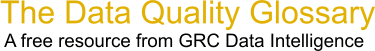Choose Index below for a list of all words and phrases defined in this glossary.

# Defects per Unit (DPU)

index | Index

## Defects per Unit  - DPU - definition(s)

Defects per Unit  - DPU - DPU or Defects Per Unit is the average number of defects observed when sampling a population.

DPU = Total # of Defects / Total population

Consider 100 electronic assemblies going through a functional test. If 10 of these fail the first time around, we have a first pass yield of 90%. Let's say the 10 fails get reworked and re-tested and 5 pass the second time around; the 5 remaining fails pass on the third attempt. Feel free to work out how this would look as a rolling yield. (100 'passes'/115 tests).

DPU takes a fundamentally different approach to the traditional measurement of yield. It is simply a ratio of the number of defects over the number of units tested (don't worry about how many tests or how many opportunities for defects).

In the above example, the DPU is 15/100 or 0.15. There are 100 units which were found to have a cumulative total of 15 defects when tested.

One interesting feature of DPU is that if you have sequential test nodes, i.e. if the above 100 units had to go through 'Final Test' and threw up a DPU figure of 0.1 there, you simply add the DPU figures from both nodes to get the overall DPU of 0.25 (this is telling you that there were 25 defects in your 100 units). There are a few assumptions which must be realised for this statement to be wholly accurate, but there isn't really time to go there in a 'definition' space.

If out of the 100 loans applications there are 30 defects, the FTT yield is .70 or 70 percent. Further investigation finds that 10 of the 70 had to be reworked to achieve that yield so our Rolled Throughput Yield is 100-(30+10)/100 = .6 or 60 percent yield.

To consider the defects per unit in this process we divide the number of defects by the result of multiplying the sample by the number of opportunities in each item.

No.of defects/(no. of units)(no. of opportunities for a defect)= 30/1003 = 30/300 = .1 or we would say that there is a 10 percent chance for a defect to occur in this process.

[Category=Data Quality ]

Source: iSixSigma, 14 January 2011 09:23:14, https:web.archive.org/web/20111109014246/http:www.isixsigma.com/index.php?option=com_glossaryData Quality Glossary.  A free resource from GRC Data Intelligence. For comments, questions or feedback: dqglossary@grcdi.nl